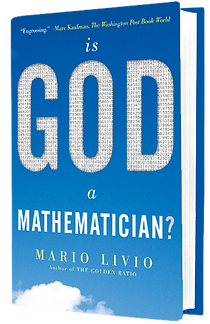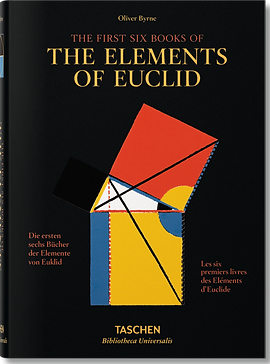top of page

# Math Fan Content

## Long Term Projects, Researchs

Mystery Mathematician "?"

Until 17th century algebra and geometry were two distinct branches of mathematics.

He was the first man who combines algebra and geometry to provide a great tool for visualizing equations with two variables.

Name this famous philosopher and mathematician.

His middle name is given to Coordinate Plane

There are different stories about how he discovered the analytic geometry. Search about them.

Introduce his findings and philosophy.

Introduce the mathematical concept and equations.

Write a few examples of his famous quotes.

He is one of the other famous mathematicians who is also a very well-known Philosopher.

Can you name others?

What do you think, why are so many philosophers also mathematicians?

Useful resources:and 2Impossible Objects - How to make them possible?

What is an impossible object?

Do you see any impossible objects around you?

-bottles with no insides (or outsides), one-edged loops, solid ball with no fixed size.

Can you make any impossible objects by using only a piece of paper and glue?

Have you ever heard about Mobius Strip?

Who discovered it?

Watch the video and learn about the original name of the shape?

Why this shape is so special?

Repeat the same magical moves to create a four-twisted loop, a square and the hearts as you have seen in the video.

Start your loop with a single twist. Make your prediction before unravelling the pieces–how many pieces will there be after halving horizontally? Will they be all the same size? How many twists will they have? Then cut it again. Answer the same questions.

Then, search about the recycling logo. Who has designed it and when? Find out the origins of this logo.

Useful resources: 1Mathematics and Philosophy

What is mathematics? How do YOU define it?

Is math science or art?

Is math invented or discovered?

Read the book “Is God a Mathematician by Mario Livio

Watch the by jeff Dekofsky.

Find the famous mathematicians and their supporting arguments for these famous debates

What do you think, why are so many philosophers also mathematicians?Image is taken from the Authors' website https://www.mario-livio.com/books

Infinity and  Far Beyond

What is infinity? Give examples to infinite things?

Can you make operations with infinity?

How many natural numbers are there?

How many evens?

How many rational numbers are there between 0 and 1? What about between 0 and 2?

So is one infinity bigger than another? Search about famous mathematician Cantor and his approach on the cardinality of the number sets

What is the history of infinity? Invented or Discovered? Who has found its symbol?

Suggested reading: Beyond Infinity by Eugenia Cheng

Math and Infinity by Ali NesinDo prime numbers have primary importance?

Is 1 a prime number? Are there more composite or prime numbers between 1-10?

Is there a pattern among the prime numbers?

Interesting facts about primes? ( ex: between a number and its double there is always a prime number)

List the methods to find primes.

Search about Goldbach Conjecture.  Explain it by giving examples

What are the other famous conjectures and theorems about prime numbers?

Visit https://www.mersenne.org to join internet’s biggest Mersenne Prime Search.

What is the largest known prime? Who , when and how was it found?

Watch the videos of Standupmaths videos by Matt Parker about prime numbers on YouTube.

Where do we use prime numbers in our daily life? Why are they so important?Euclidian Geometry... Wait! There are other geometries?!

Search about the origins of the Geometry?

Who can be named as the father of geometry? Search about the plane Geometry?

What are the basic axioms of plane geometry?

Most of the Ancient Philosophers were also great mathematicians who studied the basic concepts of Geometry. Name a few of them.

Additional search: focus on famous painting of Raphael    “The School of Athens” give information about the mathematicians pictured in this masterpiece.

Give examples of famous quotes about the relation between philosophy and geometry.

Who has found the non-euclidian geometry?

Find some demonstrations on internet

Bring a spherical balloon or ball to your presentation to make a demo of non-Euclidian Geometry.Euclid's Elements

Image is taken from the taschen.com

Iconic Number of Math

It is not possible to write it as a ratio of two integers yet it is the ratio circle’s circumference to its diameter.

People have calculated the first 10 trillion digits of it, though for most purposes – such as designing a building or sending a spacecraft to Mars.

Search about the different calculation methods of this number

• Calculate it by using infinite series

• Use Buffon’s Needle Problem

• Use the polygons method of the Ancient Greek mathematician Archimedes

• By playing Dart (1)

• Or with a pendulum (2)

• Look up the most famous rivers on earth. Calculate the ratio of the river's actual length to the distance from its source to its mouth as the crow flies.  Ready to be surprised! You can find yourself discussing whether math is an invention or discovery!  (3)

• Check the websitevideo for the artistic perspective of this fascinating number.